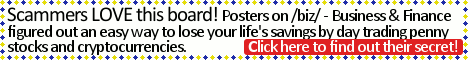[a / b / c / d / e / f / g / gif / h / hr / k / m / o / p / r / s / t / u / v / vg / vr / w / wg] [i / ic] [r9k / s4s / vip / qa] [cm / hm / lgbt / y] [3 / aco / adv / an / asp / bant / biz / cgl / ck / co / diy / fa / fit / gd / hc / his / int / jp / lit / mlp / mu / n / news / out / po / pol / qst / sci / soc / sp / tg / toy / trv / tv / vp / wsg / wsr / x] [Settings] [Search] [Home]
Board
/sci/ - Science & Math

You cannot reply anymore.$\text{Given a finite string }S\text{ of symbols }X\text{ and }O\text{, we write }\Delta(S)\text{for the number of } \\ X\text{'s in }S\text{ minus the number of }O\text{'s. For example, }\Delta(XOOXOOX) = -1\text{. We} \\ \text{call a string }S\textbf{ balanced}\text{ if every substring }T\text{ of (consecutive symbols of) }S \\ \text{has }-2 \leq \Delta(T) \leq 2\text{. Thus, }XOOXOOX\text{ is not balanced, since it contains the} \\ \text{substring }OOXOO\text{. Find, with proof, the number of balanced strings of length} \\ n\text{.}$
>>
>>
>>10468674
Define a partial string of S as a substring that contains the first k elements of S, where k is some natural number such that 1<k<n. A string S is balanced iff the maximum delta of the partial strings of S minus the minimum delta of the partial strings of S is between -2 and 2. The formula should be 2 * (1 + sum from i = 2 to n of 2^(ceil((n - i - 1)/2))). At the first part of the algorithm, you either pick X or O, and then you fill in with alternating symbols; you continue until i - 1 terms of the sequence have been filled in. i represents the part of the sequence where you duplicate the previous term, however, after duplication, you must pick the opposite symbol, and, from there, you may start filling in the rest of the sequence with either XOs, or OXs, with possibly a X or an O at the end of the sequence.
>>
bump
>>
>>10468674
S cannot contain a substring of the form XX((OX)^n)X or OO((XO)^n)O
>>
thinly veiled homework thread
>>
>>10470376
Use regular expressions to (carefully) uniquely generate all strings that aren't accepted by the DFA
The terms that stop at S are e (the empty string)
... at A are A(BA)* + B(AB)*A
... at B are B(AB)* + A(BA)*B
... at AA are [ A(BA)*A + B(AB)*AA + ( B(AB)*B + A(BA)*BB )(AB)*AA ][ (BA)*BB(AB)*AA ]*
... at BB are [ B(AB)*B + A(BA)*BB + ( A(BA)*A + B(AB)*AA )(BA)*BB ][ (AB)*AA(BA)*BB ]*
... at A_ are [ B(AB)*B + A(BA)*BB + ( A(BA)*A + B(AB)*AA )(BA)*BB ] (AB)*A [ A(BA)*BB(AB)*A ]*
... at B_ are [ A(BA)*A + B(AB)*AA + ( B(AB)*B + A(BA)*BB )(AB)*AA ] (BA)*B [ B(AB)*AA(BA)*B ]*

Converting these to generating functions is pretty easy.
at S ~ 1
at A ~ (t+t^2)/(1-t^2)
at B ~ (t+t^2)/(1-t^2)
at AA ~ [ (t^2 + t^3)/(1-t^2) + (t^2 + t^3)/(1-t^2) * (t^2)/(1-t^2) ] * [ 1/( 1 - (t^4)/( 1-t^2 )^2 ) ]
at BB ~ [ (t^2 + t^3)/(1-t^2) + (t^2 + t^3)/(1-t^2) * (t^2)/(1-t^2) ] * [ 1/( 1 - (t^4)/(1-t^2 )^2 ) ]
at A_ ~ [ (t^2 + t^3)/(1-t^2) + (t^2 + t^3)/(1-t^2) * (t^2)/(1-t^2) ] * (t/(1-t^2)) * [ 1/( 1 - (t^4)/( 1-t^2 )^2 ) ]
at B_ ~ [ (t^2 + t^3)/(1-t^2) + (t^2 + t^3)/(1-t^2) * (t^2)/(1-t^2) ] * (t/(1-t^2)) * [ 1/( 1 - (t^4)/( 1-t^2 )^2 ) ]

Adding all of these terms yields (2t^3 + 2t^2 + 2t + 1))/(1-2t^2)
Which gives (3t + 2)/(1 - 2t^2) - t - 1

If n=0, the coefficient of t^n is 1 (the empty string)
If n=1, the coefficient of t^n is 2
If n>=2 is even, the coefficient of t^n is 2^(n/2 + 1)
If n>=3 is odd, the coefficient of t^n is 3*2^((n-1)/2)

I might be a robot.
>>
I'm gonna save this problem with the intention of solving it when I have time and never look at it again.
>>
>>10470893
I messed up somewhere.
Fuck the regexps.
>>10470376
Just let the nodes represent generating functions and compute them according to which nodes point to a given node.
Let S=1 and A=B, A2 = B2, A_ = B_ by symmetry.
A = St + Bt = t + At so A = t/(1-t)
A2 = At + (A_)t + (B_)t = t(A + 2A_)
A_ = (A2)t

A2 = At + 2(A2)t^2
A2 = At/(1-2t^2) = (t^2)/((1-t)(1-2t^2))

S + A + B + A2 + B2 + A_ + B_ = 1 + 2( t/(1-t) + (t^2 + t^3)/((1-t)(1-2t^2)) )
= (4t + 3)/(1 - 2t^2) - 2/(1-t)

n even gives 3*2^(n/2) - 2
n odd gives 4*2^((n-1)/2) - 2

Delete Post: [File Only] Style:
All trademarks and copyrights on this page are owned by their respective parties. Images uploaded are the responsibility of the Poster. Comments are owned by the Poster.